# 例子描述

An athlete’s performance and the number of his trainings are as follows: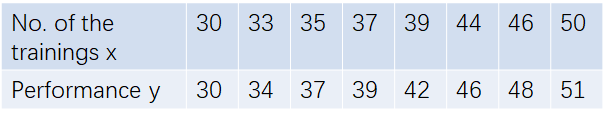1)Plot the data
2)Write a program to fit the linear regression parameters ? to the data using gradient descent
3)Predict his performance in the 47th and the 55th training

# 梯度下降法简介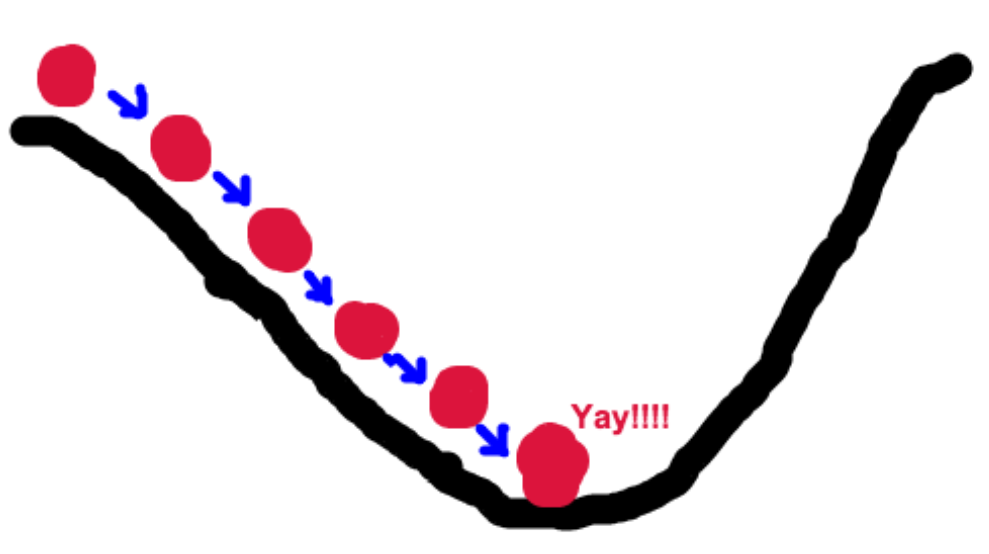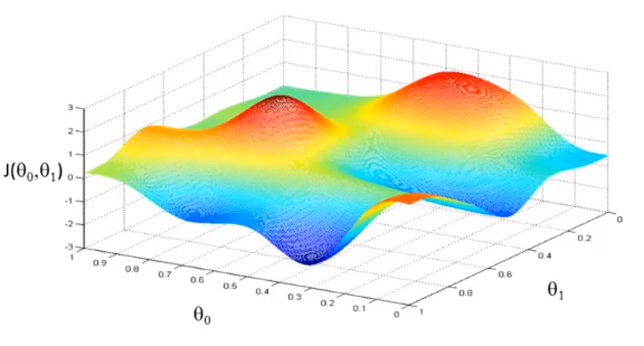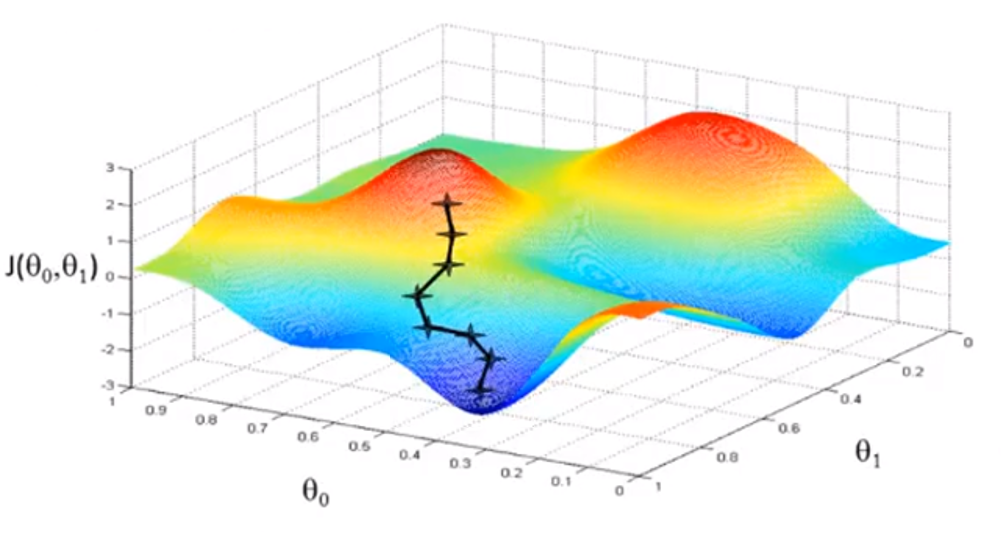ok，现在我们已经知道不断沿着梯度可以找到极值点了（对于凸函数是最值点）！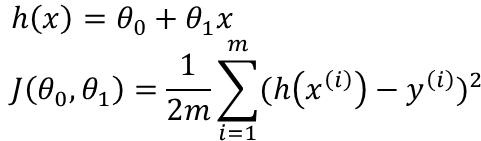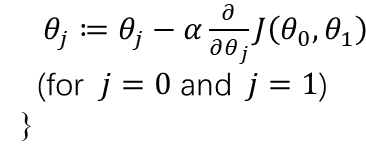[雾 ٩( ‘ω’ )و

# 基于python3 matplotlib的实现代码

## 梯度下降法实现

import numpy as np
import matplotlib.pyplot as plt

N = 8
x = np.array([30,33,35,37,39,44,46,50]).reshape(N,1)
y = np.array([30,34,37,39,42,46,48,51]).reshape(N,1)
plt.scatter(x,y,color="orange",linewidth=0.5)
ones = np.ones((N, 1))
x = np.hstack((x, ones))

def CostFunction(theta, x, y):
diff = np.dot(x, theta) - y
loss = (1./2*N)*(np.dot(np.transpose(diff), diff))
return loss

def CostGradient(theta, x, y):
diff = np.dot(x, theta) - y
gradient = (1./N)*(np.dot(np.transpose(x), diff))
return gradient

def Iteration():
alpha = 0.001
theta = np.array([1, -0.1]).reshape(2, 1)
gradient = CostGradient(theta, x, y)
epsilon = 0.001
while np.linalg.norm(gradient) > epsilon:
theta = theta - alpha * gradient
gradient = CostGradient(theta, x, y)
return theta

Right_theta = Iteration()
print('下降后θ值为：')
print('θ(0)=',Right_theta,'θ(1)=',Right_theta)
print('拟合函数为:')
print('y=',Right_theta,'+',Right_theta,'x')
print('预测第47次训练后，运动员表现：',Right_theta+Right_theta*47)
print('预测第55次训练后，运动员表现：',Right_theta+Right_theta*55)

x1 = np.linspace(25, 55, 100)
y1 = Right_theta*x1 + Right_theta
plt.plot(x1, y1,color="black",linewidth=0.5)
plt.rcParams['figure.figsize'] = (8.0, 8.0)
plt.show()


## 最小二乘法实现

import numpy as np
import scipy as sp
import matplotlib.pyplot as plt
from scipy.optimize import leastsq

x = np.array([30,33,35,37,39,44,46,50])
y = np.array([30,34,37,39,42,46,48,51])
plt.scatter(x,y,color="orange",linewidth=0.5)

def func(p,x):
k,b=p
return k*x+b

def error(p,x,y):
return func(p,x)-y

p0=[1, -0.1]
Para=leastsq(error,p0,args=(x,y))
k,b=Para

print('最小二乘法求解系数：')
print('k=',k,'b=',b)
print('拟合函数为:')
print('y=',b,'+',k,'x')
print('预测第47次训练后，运动员表现：',b+k*47)
print('预测第55次训练后，运动员表现：',b+k*55)

x1 = np.linspace(25, 55, 100)
y1 = k*x1+b
plt.plot(x1, y1,color="black",linewidth=0.5)
plt.rcParams['figure.figsize'] = (8.0, 8.0)
plt.show()


# 比较结果&总结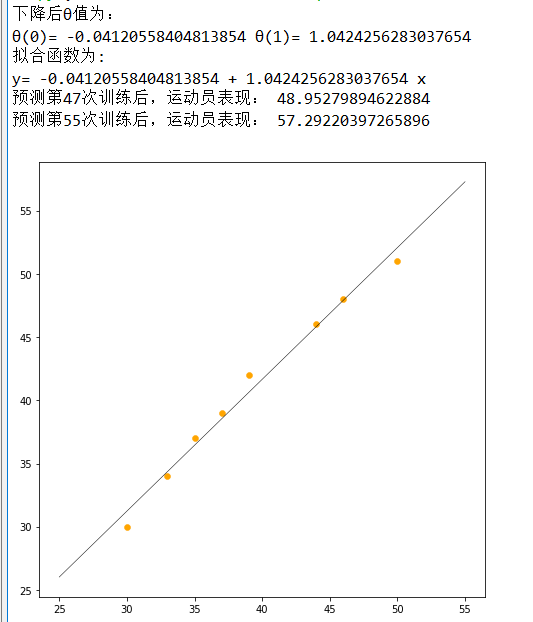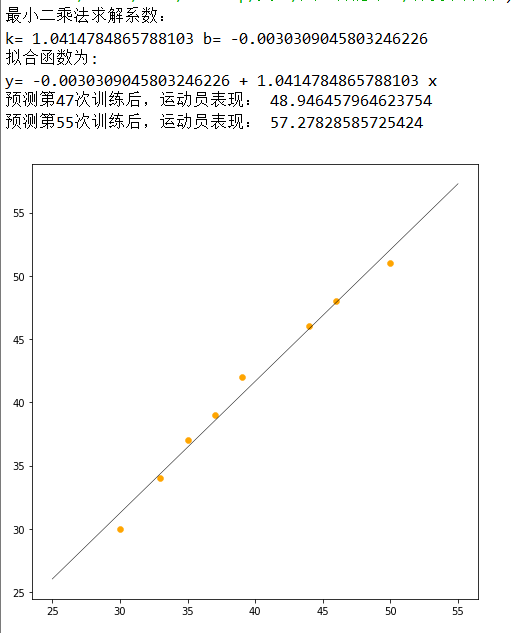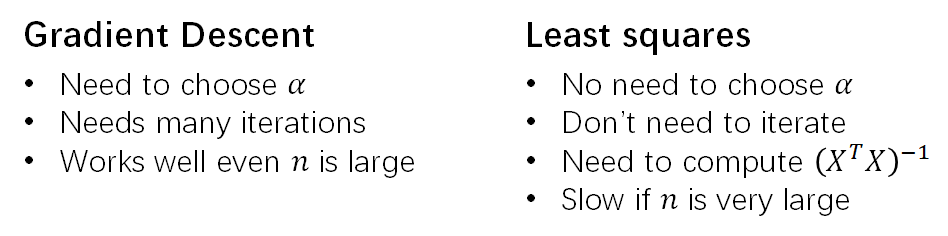CSDN首篇，AI本科在读，欢迎大佬来指出错误。

02-1504-264916
06-015万+
03-13310
01-01
03-22956
06-111万+
02-158083
05-151838
12-021630
11-17405
03-12205
11-27640
01-186107
08-055993
08-181万+
12-072736
05-029455
09-121147
©️2020 CSDN 皮肤主题: 游动-白 设计师:白松林